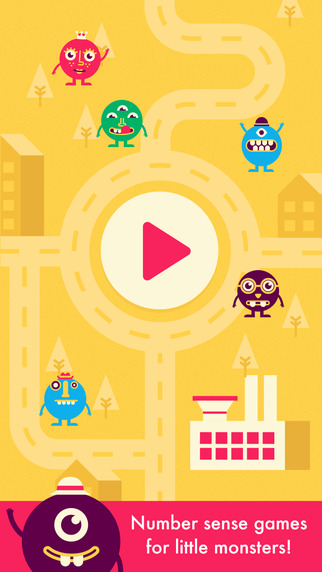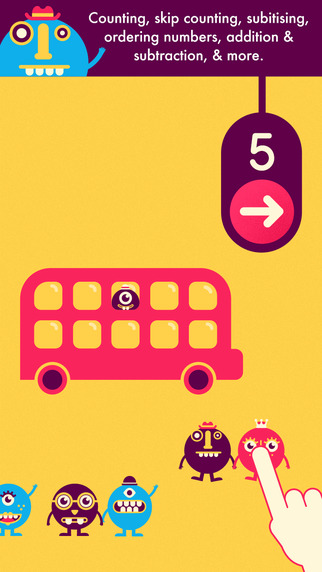EducationQuick Maths Jr.  +  iPhone app / iPad app 雙系統版本Optimized for iPhone5Free for a limited time as part of the App Store's Great Australian Apps and Games promotion!

Perfect for children aged 3 - 7, Quick Math Jr. covers important foundations of mathematics including counting, addition and subtraction, place value, writing numbers and much more! As children play through the SIX fun games, Quick Math Jr. dynamically adjusts the difficulty of questions so that young learners are always at a challenge level that is just right for them! From the creators of the award-winning Quick Math series, Quick Math Jr. is a fun way to develop mathematics skills and confidence.

FEATURES
• Six games aligned with international maths curriculums, including US Common Core and the Australian National Curriculum
• Adaptive difficulty to quickly place players at the appropriate level, with challenges to keep them in their zone of proximal development.
• Write answers directly on-screen! Players progress from multiple-choice to handwritten answers.
• Create your own monster characters by winning new features, then see your creations appear in the game!
• Unlimited player profiles for easy device sharing

EDUCATIONAL CONTENT
• Develop number sense
• Name numbers
• Practice number sequence up to 100
• Connect number names, numerals and quantities up to 30, including zero
• Model numbers up to 20
• Subitise small collections of objects in dice patterns, ten-frames and random patterns
• Conceptually subitise larger collections of objects in two or more dice patterns or ten frames
• Start counting from numbers other than one
• Count on from numbers other than one
• Skip count by twos, threes, fives and tens
• Count and skip-count forwards and backwards
• Practice place value by composing numbers to 99 using tens and ones
• Create equality using balance
• Add to and subtract from a hidden total to practice mental arithmetic and counting on and back
• Solve simple addition and subtraction questions up to 20
• Practice writing numerals

YEAR 1
Number - number and place value
Pupils should be taught to:
• count to and across 100, forwards and backwards, beginning with 0 or 1, or from any given number
• count, read and write numbers to 100 in numerals; count in multiples of twos, fives and tens
• identify and represent numbers using objects and pictorial representations including the number line, and use the language of: equal to, more than, less than (fewer), most, least
• read and write numbers from 1 to 20 in numerals and words.
Number - addition and subtraction
Pupils should be taught to:
• read, write and interpret mathematical statements involving addition (+), subtraction (–) and equals (=) signs
• add and subtract one-digit and two-digit numbers to 20, including zero

YEAR 2
Number - number and place value
Pupils should be taught to:
• count in steps of 2, 3, and 5 from 0, and in tens from any number, forward and backward
• recognise the place value of each digit in a two-digit numbers (tens, ones)
• identify, represent and estimate numbers using different representations, including the number line
• read and write numbers to at least 100 in numerals and in words
Number - addition and subtraction
Pupils should be taught to:
• recall and use addition and subtraction facts to 20 fluently, and derive and use related facts up to 100
• add and subtract numbers using concrete objects, pictorial representations, and mentally, including:
• a two-digit number and ones
• a two-digit number and tens
• two two-digit numbers